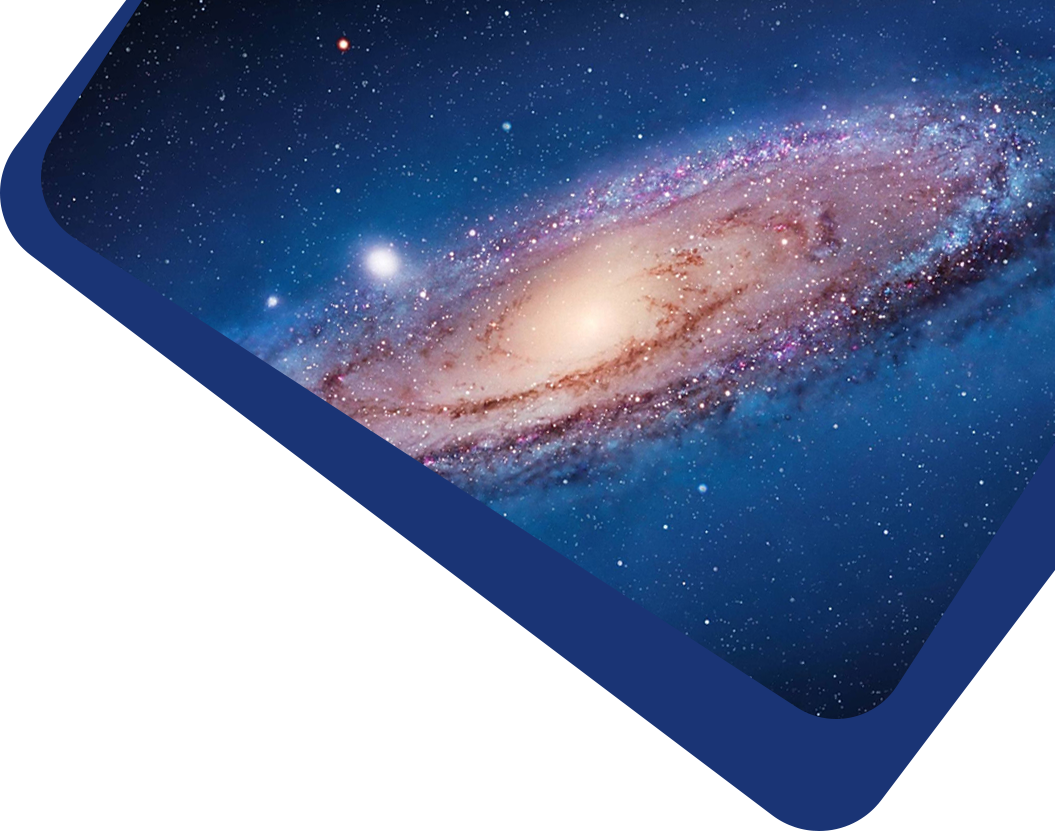Vol 20, No 8

## Inversion of Venus internal structure based on geodetic data

#### Chi Xiao, Fei Li, Jian-Guo Yan, Wei-Feng Hao, Yuji Harada, Mao Ye, Jean-Pierre Barriot

Abstract

Abstract Understanding the internal structure of Venus promotes the exploration of the evolutionary history of this planet. However, the existing research concerning the internal structure of Venus has not used any inversion methods. In this work we employed an inversion method to determine the internal structure of Venus using observational or hypothetical geodetic data; these data include mass, mean radius, mean moment of inertia and second degree tidal Love number $$k_2$$. To determine the core state of Venus, we created two models of Venus, an isotropic 3-layer model with entire liquid core and an isotropic 4-layer model with liquid outer core and a solid inner core, assuming that the interior of Venus is spherically symmetric and in hydrostatic equilibrium. A series of the sensitivity analysis of interior structure parameters to the geodetic data considered in here shows that not all of the parameters can be constrained by the geodetic data from Venus. On this basis, a Markov Chain Monte Carlo algorithm was used to determine the posterior probability distribution and the optimal values of the internal structure parameters of Venus with the geodetic data. We found that the 3-layer model is more credible than the 4-layer model via currently geodetic data. For the assumption of the 3-layer model with the $$k_2 = 0.295 \pm 0.066$$, $$I/MR^2 = 0.33 ± 0.0165$$, and $$\bar{\rho} = 5242.7 \pm 2.6$$ kg m −3 , the liquid iron-rich core of Venus has a radius of $$3294^{+215}_{−261}$$ km, which suggests a larger core than previous research has indicated. The average density of the mantle and liquid core of Venus are $$4101^{+325}_{-375}$$ and $$11885^{+955}_{-1242}$$ kg m-3, respectively.

Keywords

Keywords planets and satellites: interiors — planets and satellites: terrestrial planets — planets and satellites: physical evolution — planets and satellites: fundamental parameters

Full Text
Refbacks

• There are currently no refbacks.